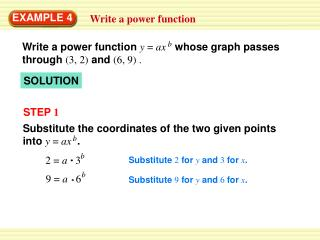DownloadDownload PresentationEXAMPLE 4

# EXAMPLE 4

Télécharger la présentation## EXAMPLE 4

- - - - - - - - - - - - - - - - - - - - - - - - - - - E N D - - - - - - - - - - - - - - - - - - - - - - - - - - -
##### Presentation Transcript

1. b Write a power function y = axwhose graph passes through (3, 2) and (6, 9) . Substitute the coordinates of the two given points into y = ax. b b 2 = a 3 b 9 = a 6 EXAMPLE 4 Write a power function SOLUTION STEP 1 Substitute 2 for yand 3 forx. Substitute 9 for yand 6 forx.

2. Solve for ain the first equation to obtain a= , and substitute this expression for ain the second equation. Log 4.5 2 Substitute for ain second equation. b 9 = 6 b Log2 3 2 3 b Log 4.5 = b 2 b Take log of each side. 2 9 = 2 2 b 4.5 = 2 2 3 b = b 2.17 b EXAMPLE 4 Write a power function STEP 2 Simplify. Divide each side by 2. Change-of-base formula Use a calculator.

3. 2 3 2.17 2.17 Determine that a = 0.184. So, y = 0.184x. EXAMPLE 4 Write a power function STEP 3

4. b Write a power function y = ax whose graph passes through the given points. for Example 4 GUIDED PRACTICE 5. (2, 1), (7, 6) 1.43 y = 0.371x SOLUTION 6. (3, 4), (6, 15) 1.91 y = 0.492x SOLUTION 7. (5, 8), (10, 34) 2.09 y = 0.278x SOLUTION

5. for Example 4 GUIDED PRACTICE 8.REASONINGTry using the method of Example 4 to find a power function whose graph passes through (3, 5) and (3, 7). What can you conclude? SOLUTION The points cannot form a power function.